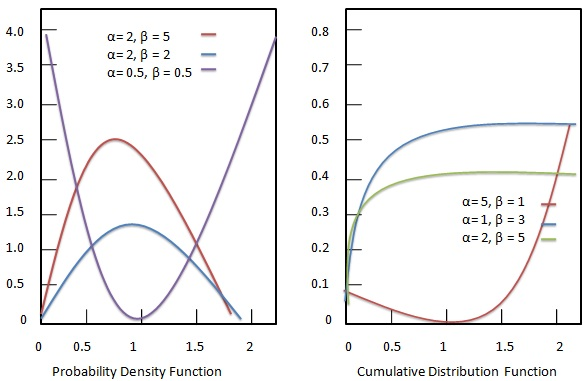# Statistics - Beta Distribution

The beta distribution represents continuous probability distribution parametrized by two positive shape parameters, $\alpha$ and $\beta$, which appear as exponents of the random variable x and control the shape of the distribution.### Probability density function

Probability density function of Beta distribution is given as:

## Formula

${ f(x) = \frac{(x-a)^{\alpha-1}(b-x)^{\beta-1}}{B(\alpha,\beta) (b-a)^{\alpha+\beta-1}} \hspace{.3in} a \le x \le b; \alpha, \beta > 0 \\[7pt] \, where \ B(\alpha,\beta) = \int_{0}^{1} {t^{\alpha-1}(1-t)^{\beta-1}dt} }$

Where −

• ${ \alpha, \beta }$ = shape parameters.

• ${a, b}$ = upper and lower bounds.

• ${B(\alpha,\beta)}$ = Beta function.

### Standard Beta Distribution

In case of having upper and lower bounds as 1 and 0, beta distribution is called the standard beta distribution. It is driven by following formula:

## Formula

${ f(x) = \frac{x^{\alpha-1}(1-x)^{\beta-1}}{B(\alpha,\beta)} \hspace{.3in} \le x \le 1; \alpha, \beta > 0}$

### Cumulative distribution function

Cumulative distribution function of Beta distribution is given as:

## Formula

${ F(x) = I_{x}(\alpha,\beta) = \frac{\int_{0}^{x}{t^{\alpha-1}(1-t)^{\beta-1}dt}}{B(\alpha,\beta)} \hspace{.2in} 0 \le x \le 1; p, \beta > 0 }$

Where −

• ${ \alpha, \beta }$ = shape parameters.

• ${a, b}$ = upper and lower bounds.

• ${B(\alpha,\beta)}$ = Beta function.

It is also called incomplete beta function ratio.